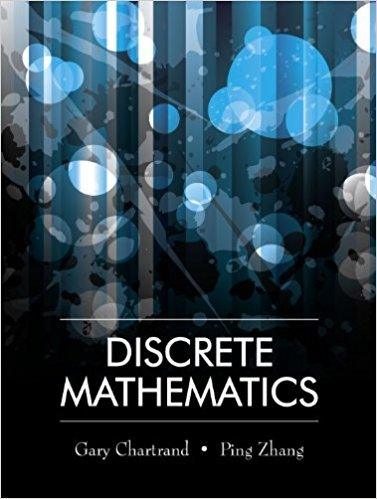×
×

# Solutions for Chapter 5.5: Cardinalities of Sets## Full solutions for Discrete Mathematics | 1st Edition

ISBN: 9781577667308Solutions for Chapter 5.5: Cardinalities of Sets

Solutions for Chapter 5.5
4 5 0 302 Reviews
24
1
##### ISBN: 9781577667308

This expansive textbook survival guide covers the following chapters and their solutions. This textbook survival guide was created for the textbook: Discrete Mathematics, edition: 1. Since 15 problems in chapter 5.5: Cardinalities of Sets have been answered, more than 13840 students have viewed full step-by-step solutions from this chapter. Discrete Mathematics was written by and is associated to the ISBN: 9781577667308. Chapter 5.5: Cardinalities of Sets includes 15 full step-by-step solutions.

Key Math Terms and definitions covered in this textbook
• Cholesky factorization

A = CTC = (L.J]))(L.J]))T for positive definite A.

• Companion matrix.

Put CI, ... ,Cn in row n and put n - 1 ones just above the main diagonal. Then det(A - AI) = ±(CI + c2A + C3A 2 + .•. + cnA n-l - An).

• Complete solution x = x p + Xn to Ax = b.

(Particular x p) + (x n in nullspace).

• Cramer's Rule for Ax = b.

B j has b replacing column j of A; x j = det B j I det A

• Determinant IAI = det(A).

Defined by det I = 1, sign reversal for row exchange, and linearity in each row. Then IAI = 0 when A is singular. Also IABI = IAIIBI and

• Dot product = Inner product x T y = XI Y 1 + ... + Xn Yn.

Complex dot product is x T Y . Perpendicular vectors have x T y = O. (AB)ij = (row i of A)T(column j of B).

• Eigenvalue A and eigenvector x.

Ax = AX with x#-O so det(A - AI) = o.

• Full row rank r = m.

Independent rows, at least one solution to Ax = b, column space is all of Rm. Full rank means full column rank or full row rank.

• Indefinite matrix.

A symmetric matrix with eigenvalues of both signs (+ and - ).

• Left inverse A+.

If A has full column rank n, then A+ = (AT A)-I AT has A+ A = In.

• Network.

A directed graph that has constants Cl, ... , Cm associated with the edges.

• Normal matrix.

If N NT = NT N, then N has orthonormal (complex) eigenvectors.

• Orthonormal vectors q 1 , ... , q n·

Dot products are q T q j = 0 if i =1= j and q T q i = 1. The matrix Q with these orthonormal columns has Q T Q = I. If m = n then Q T = Q -1 and q 1 ' ... , q n is an orthonormal basis for Rn : every v = L (v T q j )q j •

• Permutation matrix P.

There are n! orders of 1, ... , n. The n! P 's have the rows of I in those orders. P A puts the rows of A in the same order. P is even or odd (det P = 1 or -1) based on the number of row exchanges to reach I.

• Random matrix rand(n) or randn(n).

MATLAB creates a matrix with random entries, uniformly distributed on [0 1] for rand and standard normal distribution for randn.

• Rank one matrix A = uvT f=. O.

Column and row spaces = lines cu and cv.

• Reflection matrix (Householder) Q = I -2uuT.

Unit vector u is reflected to Qu = -u. All x intheplanemirroruTx = o have Qx = x. Notice QT = Q-1 = Q.

• Simplex method for linear programming.

The minimum cost vector x * is found by moving from comer to lower cost comer along the edges of the feasible set (where the constraints Ax = b and x > 0 are satisfied). Minimum cost at a comer!

• Solvable system Ax = b.

The right side b is in the column space of A.

• Transpose matrix AT.

Entries AL = Ajj. AT is n by In, AT A is square, symmetric, positive semidefinite. The transposes of AB and A-I are BT AT and (AT)-I.

×